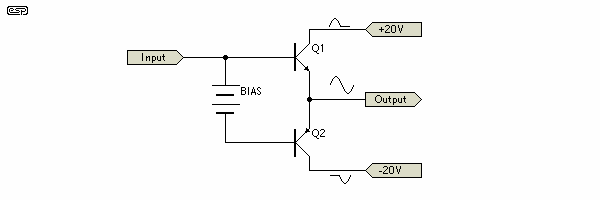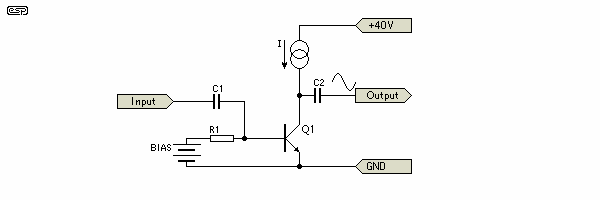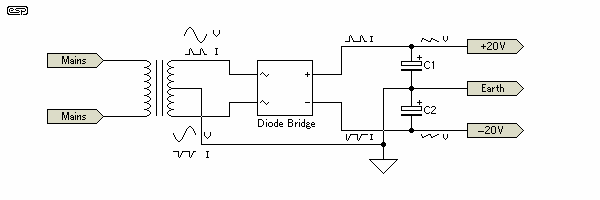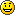Elliott Sound Products Power Amplifier Efficiency Explained

Power Amplifier Efficiency Explained

© 1999, Rod Elliott (ESP)
Page Last Updated 29 Jan 2000Main IndexArticles Index

Contents
Introduction

Amplifier efficiency is a topic that seems to be poorly understood, judging from the comments and questions I have had from readers, so I thought that I would put something together to explain away some of the mystery.  As I progressed, it became obvious that I was going to have a lot more work to do than originally intended, since many of the basic concepts will also require explanation.

Efficiency in itself is not hard - power out versus power in.  No electronic device can ever be 100% efficient, and all lost power in an amplifier is converted to heat.  It makes some sense to try to make an amp as efficient as possible, but if this causes an unacceptable degradation of the sound quality, then it matters not how many efficiency stars it has, no-one will want to listen to it.

At the other end of the scale, there are Class-A amplifiers, some of which are so inefficient that it almost defies belief.  This waste of power does not necessarily translate into better sound, although reading some material would lead you to think that the lower the efficiency, the better it must sound.  This is (of course) not true.

This article describes a single channel amplifier, using Class-A and Class-B.  No allowance has been made for reactive loads (as occur in loudspeakers), which can increase power dissipation dramatically.

Amplifier Power Losses

So, where do the power losses come into play?  We will need to look at a basic amplifier design first, so that the mechanism can be seen clearly.  I shall start with a Class-B stage, as these are the most common (although very few are truly Class-B, most are actually Class-AB - but more on this later).  For all calculations, a sinewave is the signal source, and for those who might be ready to claim that music is much more complex, music - and indeed any waveform - is derived entirely from sinewaves of differing frequencies and amplitudes.Figure 1 - An Ideal Class-B Amplifier

Class-B

We will start with the assumption that the transistors in Figure 1 are 'ideal', in that there is no base current needed (they have infinite gain), and no voltage at all is lost when fully conducting.  The bias voltage is adjusted so that the transistors are exactly on the verge of conduction, but no quiescent current flows.  Given that the supply voltage is +/-20V, this allows a peak swing into an ideal 8 Ohm resistive load of 20 Volts, which is an RMS voltage of 14.14V.  The peak current is 20/8, or 2.5 Amps, and this equates to 1.768 Amps RMS.  The power to the load is 25 Watts.

P = V * I     or ...
P = V² / R     or ...
P = I² * R

Where P = power in Watts, V = voltage, I = current, and R = resistance.  Voltage and current are RMS.  Note that the term "RMS Power" is erroneous - power is the result of RMS voltage and RMS current applied to a load, and is measured in Watts.  Although "RMS power" is not real, it has become accepted to mean that RMS voltage and RMS current were used to measure the power.  Any other 'rating' (such as PMPO) is completely worthless for comparison.

The DC current waveform is shown on the diagram, and it can be seen that for each half cycle, one supply or the other supplies the current to the load.  This can safely be ignored, as RMS (with a sinewave signal) implies that  the waveform is symmetrical, and therefore the added individual DC currents make a "whole" RMS current.

The RMS input current (see note, below) must therefore equal the RMS output current, and we can now calculate the input power using the first equation above.  The DC applied is 20V, so the input power is 20 * 1.768A, or 35.36W.  The 10.36W difference is dissipated as heat in the output devices (5.18W each), and is removed using a heatsink.  We do not need to take the entire supply voltage (40V) into account, since only half of it is used at any point in time.

Efficiency may now be calculated as

Eff = Pout / Pin * 100 = 25 / 35.36 * 100 = 70.7%

This is the theoretical maximum efficiency of a Class-B push-pull amplifier, assuming no losses.  Since in real life (as opposed to advertising brochures) there are always losses, this figure can only be approached - no amplifier will equal or exceed the maximum theoretical efficiency.  Of course there are many amplifiers that use multiple power supplies, modulated DC, and many other electronic tricks, but at the end, when operating at full power they are all limited to this figure.  Class-D (switchmode or pulse-width-modulated) amplifiers can exceed this figure, but they are a completely different animal, and will not be discussed in this article.

Note:  It is worth pointing out that using RMS current for the input power calculation is convenient, but not strictly correct.  It can be shown that the average value is more accurate, and for a sinewave (the waveform of choice for all power tests) this is equivalent to 0.636 (rather than 0.707) of the peak input current.  The difference is actually not at all great, especially since the amplifier should spend virtually none of its time at maximum output power (since the likelihood of clipping is very great indeed).  It is in the interests of accuracy that I let you know that ...

Average current (using the same example as above) is 2.5A (peak) * 0.636 = 1.59A, so maximum efficiency is

Eff = Pout / Pin * 100 = 25 / 31.8 * 100 = 78.6%

The 'textbook' figure says 78.53%, so the above is close enough for our purposes.  This still assumes that there are no losses in the devices (never the case in practice), and again is only applicable at full power.  In reality, efficiency will be less, and about 70% at full power is normal for typical Class-AB amplifiers when all factors are taken into consideration.

All the above figures are quoted at maximum power, and a Class-B amp will still maintain a passable efficiency at lower powers, since the ratio of input power to output power is maintained - at least to some degree (see below).  The input to output power ratio is actually quite complex.  As power is reduced, efficiency is also reduced, and output device dissipation increases until a critical point is reached, after which it again falls with falling output power.  The table below shows the relationship, and this must be considered when designing an amplifier, so that the safe operating power levels of the devices are not exceeded.  This is based on ideal devices, so there will be about a 5% to 10% overall reduction of efficiency in real world amplifiers.  In order to take the overall losses into account, I have used the RMS input current for these calculations - although not strictly accurate, this more closely approximates the actual (as opposed to theoretical minimum) losses incurred in practical designs.

 Vout (Peak) Vout (RMS) Iout Pout Pin Dissipation (W) 20 14.14 1.77 25.00 35.36 10.36 18 12.73 1.59 20.25 31.82 11.57 16 11.31 1.41 16.00 28.28 12.28 14 9.90 1.24 12.25 24.75 12.50 12 8.49 1.06 9.00 21.21 12.21 10 7.07 0.88 6.25 17.68 11.43 8 5.66 0.71 4.00 14.14 10.14 6 4.24 0.53 2.25 10.61 8.36 4 2.83 0.35 1.00 7.07 6.07 2 1.41 0.18 0.25 3.54 3.29 1 0.71 0.09 0.06 1.77 1.71
Table 1 - Class-B Power Dissipation Versus Output

At 1/2 power (12.5W in this case), the dissipation is at a maximum, and the dissipated power exactly equals the output power, giving an efficiency of 50%.  It is also apparent that at lower powers efficiency falls even more, but output stage dissipation also falls, so heating would not seem to be such a problem.  However, since we are working with music in the real world, we need to consider the dynamic range (or, to be more precise, the peak to average ratio) of a typical signal.  This is between 10dB and 20dB, so average power will be somewhere between 2.5 and 8 Watts.  From the table we can see that the amplifier will be working fairly consistently at an average dissipation of between 4 to 10 Watts with normal programme material at the onset of clipping for a 25W amplifier.

This is not often taken into consideration, and many amplifiers have insufficient heatsink capacity to operate at anywhere near full power for prolonged periods.  Thankfully, most music is listened to at fairly moderate levels most of the time, so the problem is rarely as bad as it looks.  Sinewave testing is a most arduous test of an amplifier, quite contrary to the beliefs of some who claim that it is too simple, and cannot give any reliable indication of the amp's performance on complex music.  It is rare music indeed that will stress an amp like a sinewave test will do, as there is normally a constant variation of power allowing the heatsink time to dissipate the heat generated during loud passages.

Class-A

Class-A amps come in many 'flavours', but can be loosely separated into two separate groups.  The first (and simplest) is as shown in Figure 2, where a transistor (although it can easily be a MOSFET or valve) is simply loaded with a constant current source.  Although a resistor can be used instead of the current source, this reduces performance.  An inductor or transformer is usually used for valve Class-A amplifiers.Figure 2 - A Basic Class-A Amplifier

The bias voltage of the amp in Figure 2 must be high enough to ensure that the transistor's quiescent current is about equal to (or slightly greater than) the peak speaker current.  The efficiency of this amp can be easily calculated by the same method as described above.  A Class-A amp conducts at all times and the DC is at least equal to the peak output current.  A 20V peak signal will still create a 2.5A peak current in the load, so the DC quiescent current must be equal to (or greater than) this figure.  Note that the supply is not bipolar - a single +40V supply is the same as a ±20V supply for the purposes of calculation.  The output to the load will still be 25W, but the DC input power is now 100W (2.5A * 40V).  Efficiency now (from equation 4) is ...

Eff = 25 / 100 * 100 = 25%

This is again the best that can be expected, and there are some Class-A topologies that are considerably worse than this.  Without going into extreme detail, efficiency of this type of Class-A stage can range from about 12.5% up to about 22% (allowing an additional amount of current to ensure that the transistors never reach a point where no current flows).  The 'modulated current source' (as used the original Linsley Hood amp), can reach efficiencies up to about 30 to 35%.  With a Class-A amp, the efficiency falls with reduced power, until at zero output power, efficiency is 0%.  At an output power of 1W, efficiency is 1%, and so on (with the voltages and currents as described).

Another type of Class-A amplifier uses the same circuit as Figure 1, but the transistors are biased to around ½ the peak speaker current.  With signal, the transistors can draw up to double the quiescent current at waveform peaks, or zero at the opposite peak (one transistor will be double and the other zero as the waveform alternates).  Dynamic analysis of this arrangement is harder than either of the other topologies described, but if we examine the quiescent state and the maximum output, a reasonable estimate is possible.  Using the same values as before, we have 20V across each transistor, at a quiescent current of 1.25A.  Each transistor will therefore dissipate 25W with no signal, and maximum power is also 25W.  Efficiency is therefore ...

Eff = 25 / 50 * 100 = 50%

Again, this is the absolute maximum possible efficiency, and in general it will be lower.  How much lower depends on many factors, but one can reasonably expect the actual figure to be perhaps 40% or so at best.  The most common application of this technique is with amplifiers that claim to be Class-A over part of their maximum power, reverting to Class-AB for higher powers.  This allows the amp to operate in Class-A up to perhaps 10W or more, and the majority of listening will probably be within this range unless the loudspeakers are very inefficient.

Power Supply Losses

But wait, there's more! The power supply is expected to convert its input sinewave (at 50 or 60Hz) into a nice smooth DC that the amplifier can use.  This also has losses, since the laws of physics are omnipresent.  Since we used +/-20V as the power for the amp, we shall work with the same voltage output from the power supply, and will assume 'typical' components.

A 14.14V RMS sinewave will provide 20V when rectified using 'ideal' diodes.  In real life, there will be a voltage drop of about 1V (or more, depending on current) across each diode, and also losses in the transformer, which can be expected to have an internal resistance of about 1.0 Ohm (typical of a 120VA transformer).  The situation here is complicated by the fact that diode conduction is for only as very short period, so the current waveform is difficult to measure accurately.  We can still use basic theory to work out what happens, and Figure 3 shows a typical supply circuit and waveforms.  We will assume that the capacitance is so great that output ripple (although shown) can be considered negligible (bad news for the diodes though, since the peak current will be very high).

As a matter of interest, the only meter that will give a passably accurate reading of the current is a moving coil (average responding) type.  True RMS meters will get it (sometimes horribly) wrong.  The best is to use an oscilloscope, and calculate the true average from the waveform shape and amplitude.Figure 3 - Typical Power Supply

With the input of 14.14V AC, we will have a theoretical DC output of 20V (less the diode voltage drops), since the capacitors charge to the peak value of the RMS voltage.  If the peak output current (on each supply rail) is 2.5A as demonstrated above, then the input power must be at least equal to the output power plus any losses.  Even this configuration is open to some degree of interpretation.  Is the circuit of Figure 3 a full-wave centre-tap, or a bridge.  I consider it to be a dual full wave centre tap.

In reality, the AC transformer current can only be accurately determined using complex analysis, but the table below is useful for passably accurate results.  There are several other variations (such as choke input filters), but these are not commonly used due to the excessive cost and weight penalty, and are not shown.

 Rectifier Type Filter Type RMS Transformer Secondary Current Full Wave Centre Tap Capacitor Input 1.2 x DC Full Wave Bridge Capacitor Input 1.8 x DC
Table 2 - AC vs. DC Current 

Assuming a Class-B amp first, we have 20V peak output (14.14V RMS) at an AC current of 1.768A.  It is obvious that the DC input current must be equal to the AC load current, so using the above table we can determine the transformer secondary current using a full wave centre tap configuration as shown above

 Transformer secondary current = DC current * 1.2 = 1.768A * 1.2 = 2.12A Transformer secondary voltage = 28.28 + 2 x 1V diode voltage + 2.12V (transformer loss) = 28.28 + 2 + 2.12 = 33V (approx)

Since we have a transformer secondary voltage of 33V DC (±16.5V - somewhat shy of the expected 40V), we can calculate the power rating of the transformer as ...

P = V * I = 33 * 2.12 = 70VA

This seems like an extraordinary amount of power needed for a simple 25W amp, and in reality it is not needed.  A smaller transformer can be used, and although it will not be capable of providing enough current to fully recharge the capacitors on each cycle (causing the supply to droop), the maximum power will only be needed for relatively short periods.  The short-term duration of maximum power available is determined by the capacitance, with higher capacitance giving better performance (at least to a point).  We also have to accept that there will be resistive losses in the transformer, and there are additional resistive and junction voltage losses in the rectifier bridge.

The power lost by the transformer winding resistance and diodes is (typically) ...

(2V + 2.12V) * 2.12A = 8.73W

... which again is dissipated as heat.  These losses will decrease as amplifier power is reduced, but at full power from a little 25W amplifier we have lost about 19W in heat from the amp and its power supply.  Needless to say, this is much worse with a Class-A amplifier, as shown below (using much the same calculations as before, but at the higher current).

Transformers also have 'iron loss'.  This is power that is needed to maintain the magnetic flux in the transformer core, and is always present.  As the load is increased, the magnetising current becomes less significant, and at full power, the resistive losses in the windings are usually far greater than the iron loss.  It is the magnetising current that causes a transformer to get warm even with no connection to the secondary winding.  Typically, small transformers have greater iron and copper losses than large transformers per volt-amp of output (i.e. they are less efficient).

Class-A amplifiers operate at the full DC load all the time.  This means that for the Figure 2 example, at least 2.5A DC is needed on a continuous basis, requiring a 3A rating for the transformer secondary.  Since some extra voltage might be needed to allow for (say) a capacitance multiplier filter, we will give an extra 3V RMS each side.  This means that the AC voltage needs to be 38V centre tapped, at a continuous rating of 3A.  Using the same formula as above, this gives ...

VA = V * A = 38 * 3 = 114 VA

This would suggest that a 120VA transformer is needed.  The losses are now much higher, too, and are continuous where before they were transient.  With the 38V centre tapped transformer, we can expect about 25V DC before the filter - assuming one is used.  We still have the diode and transformer losses which will actually stay about the same, since the bigger transformer will have less resistive loss, but the diodes will have more.  To get the 20V supplies, we must drop about 5 Volts in the filter, so

P = 5V * 2.5A = 12.5W (each supply, +ve and -ve)

This has just added 25W filter dissipation and 10W rectifier and transformer loss (a total of 35W) to the already low efficiency of a Class-A amplifier.  Total amplifier input power is 135W, to get 25W of audio.  This will be greatly increased if you were to use a regulator, since the input voltage must be high enough to allow for power line droop (typically up to 10%).  I shall leave this as a reader exercise to work this outFrom the above, you can now see where the power goes to.  There are losses everywhere, and it is essential that the amplifier builder has a reasonable knowledge of losses and dissipation requirements for an amplifier before starting construction.  As many constructors have found, a Class-A amplifier is not a good option on a hot day if you don't have air-conditioning.  The combination of a Class-A amp, a very hot day and air-conditioning will certainly get your electricity meter spinning too, and is definitely not an environmentally friendly option.

For further reading, see Amp Design and Heatsink Design, both of which will assist in the final determination of the supply and heatsinking requirements for your next project.

References
1. Voltage Regulator Handbook - National Semiconductor 1982
2. Amplifier Design (ESP web page)
3. Heatsink Design (ESP web page)Articles IndexMain Index

 Copyright Notice. This article, including but not limited to all text and diagrams, is the intellectual property of Rod Elliott, and is Copyright © 1999.  Reproduction or re-publication by any means whatsoever, whether electronic, mechanical or electro- mechanical, is strictly prohibited under International Copyright laws.  The author (Rod Elliott) grants the reader the right to use this information for personal use only, and further allows that one (1) copy may be made for reference.  Commercial use is prohibited without express written authorisation from Rod Elliott.
Update Log: 29 Jan - added missing waveforms to drawings, fixed a few errors and ambiguous statements./ Page created and first published 19 Nov 1999